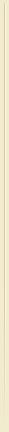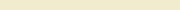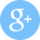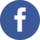STATISTICS  Consulting  and  TutoringHelp for   students Help for researchers Help for businesses Topics Courses Testimonials Rates Links Blog Art Therapy research Osteopathy research
 Are you having difficulty understanding statistics or probability? Do you need help with assignments, preparing for an exam/test, or reviewing material? Are you looking for assistance with a statistical term project or a research paper? I can help and guarantee results!
 Statistical concepts (topics) Below is the list of statistical concepts and methods that I can help you with: Qualitative and Quantitative data types [nominal, ordinal, scale/ratio, discrete and continuous variables] Grouped and Raw data Descriptive Statistics [parameter vs. statistics, central tendency and variability, mean/median/mode, standard deviation/variance, percentiles and quartiles, IQR, outliers, extreme observations] Graphical Summary [bar graphs and pie charts, histograms, box-whiskers plots, ogives, polygons] Probability [simple, conditional, marginal, union, intersection, addition and multiplication laws, independent and mutually exclusive events] Probability Distributions [uniform, binomial, hypergeometric, Poisson, normal] Sampling and Sampling Distribution [sampling techniques, SRS, Central Limit Theorem, standard error] Sample size estimation and a priori Power analysis [effect size, G*Power] Inferential Statistics and Confidence Intervals [for population mean, variance, proportion] Testing statistical significance [null and alternative hypothesis, level of significance, one and two-tailed tests, parametric and non-parametric tests, critical value and p-value approach, Type I and Type II errors, effect size, observed power] Normality testing [Kolmogorov-Smirnov and Shapiro-Wilk tests] Hypothesis Testing for one and two populations [equality of variances and Levene's F test, t-test, z-test, Welch's t-test] Repeated measures design [paired samples t-test, repeated measures ANOVA] Chi-square tests [goodness-of-fit test, test of independence] Correlation analysis [Pearson and Spearman correlation coefficients, partial correlation, testing for significance] Analysis of variance [One-way ANOVA, Two-way ANOVA, between and within factors, sphericity, simple, main and interaction effects, Repeated Measures ANOVA, ANCOVA, MANOVA] Post-hoc testing [Tukey's HSD, Scheffe, Bonferroni, Fisher's LSD] Regression analysis [simple and multiple regression, logistic and multinomial regression, variables transformation, dummy coding, multicollinearity, coefficient of determination, testing for overall and individual significance, regression equation, confidence and prediction intervals, testing assumptions, residuals] Non-parametric analysis of relationship [Chi-square, Fisher's Exact test, Phi and Cramer's V, Lambda, Gamma, Kendall's Tau] Non-parametric hypothesis testing [Mann-Whitney U test, Kruskal-Wallis ANOVA by ranks, Wilcoxon Signed-Rank test, Friedman repeated measures test] Factor/Component Analysis, Cluster and Discriminant Analysis Experimental design [controlled experiment vs observational study, external and internal validity, measurement reliability and validity, threats] Causality [spurious relationship and confounding factor, intervening variable and mediator, moderator, Sobel's z-test] Structured Equation Modeling (SEM) Linear Programming, Decision models, Optimization [Excel solver, decision tree, expected value, queuing and waiting line models] Meta-analysis of continious and categorical outcomes If you do not see the subject area or topic that you need help with, please feel free to ask.Flexible hours Available any time on weekdays, evening and weekends. Convenient locations Downtown Toronto, anywhere in GTA, or online. Contact meContact me today to solve your stats problems tomorrow! Copyright © 2009-2022 UZIK Consulting Inc.  StatsHelp ® is the registered trademark of UZIK Consulting Inc.